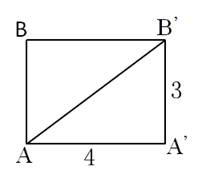### Sample Problem

A male ant is at A, the bottom of the cylinder, and sees a beautiful female ant directly above at B, the top of the cylinder. The cylinder has height 3 m and circumference 4 m. The male ant crawls completely around the cylinder to B. What is the shortest distance it must travel to get to the female ant?

m#### Solution

To find the shortest distance, we can unfold the lateral face into a rectangle. The rectangle has side lengths of the height and circumference of the cylinder, which are 3 and 4. The shortest distance between A and B is the diagonal of the rectangle, so we wish to find that length.

By the Pythagorean Theorem, we have 32 + 42 = x2, so 9 + 16 = 25 = x2 and so AB = 5 m.